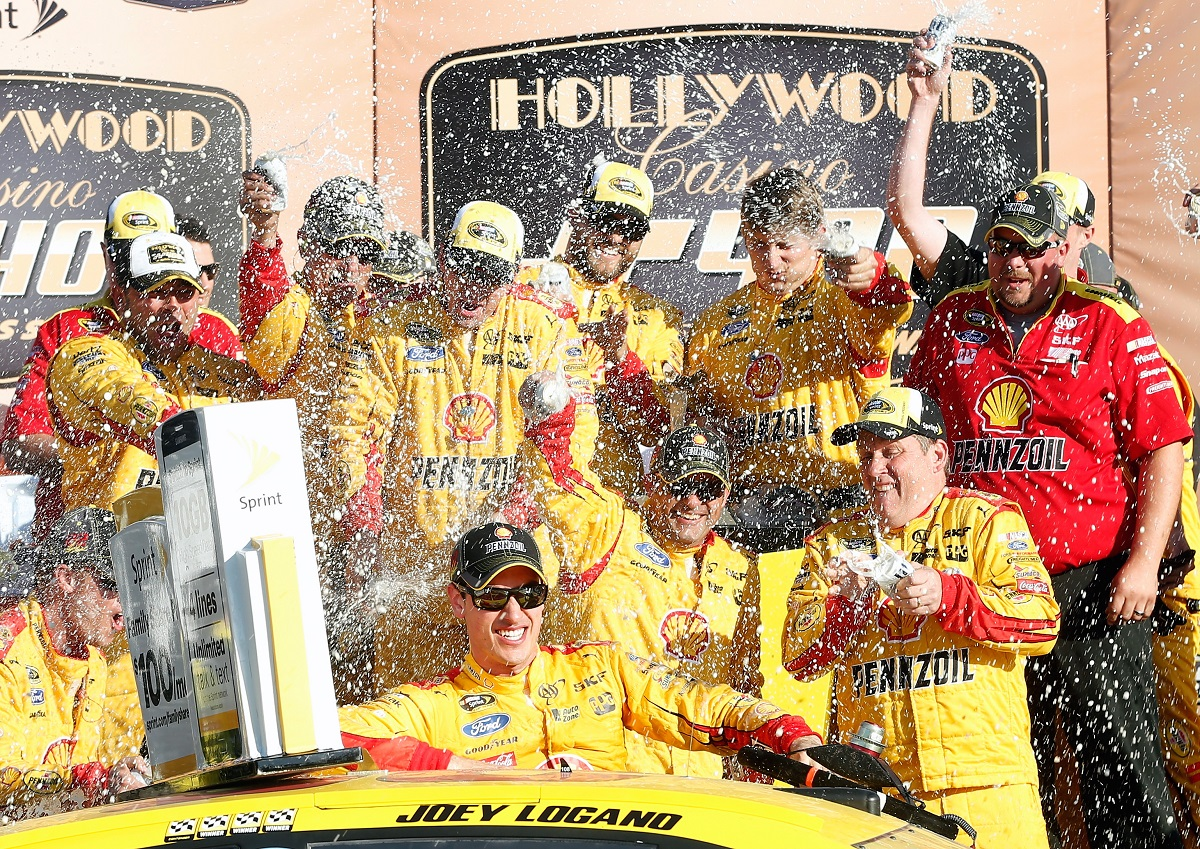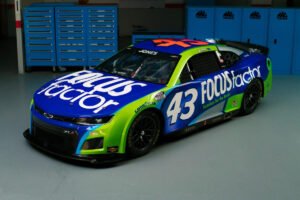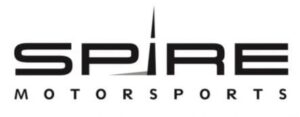November 29, 2021

# OnPitRoad.com Pick ‘Em Fantasy Pick Results for Hollywood Casino 400Photo Credit: Brain Lawdermilk/Getty Images

The Picks

Alex George and Ronald Costigan picked Kevin Harvick, Jimmie Johnson and Dale Earnhardt Jr. Harvick finished 16th with Johnson third and Earnhardt 21st. Points: 29+42+23=92

Ashley McCubbin picked Kevin Harvick, Denny Hamlin and Kyle Busch. Hamlin finished second with Busch fifth. Points: 29+42+39=110

Justin Tucker picked Brad Keselowski, Kevin Harvick and Martin Truex Jr. Keselowski finished ninth with Truex 15th. Points: 36+29+29=94

Robert Hill picked Casey Mears, Ryan Blaney and David Ragan. Mears finished 23rd with Blaney seventh with Ragan 25th. Points: 22+38+19=79

Brooke Clarke picked Kevin Harvick, Denny Hamlin and Jeff Gordon. Gordon finished 10th. Points: 29+42+34=105

Kreistina Blinova picked Kevin Harvick, Jeff Gordon and Jimmie Johnson. Points: 29+34+42=105

Marshall Gabell picked Matt Kenseth, Joey Logano and Jimmie Johnson. Kenseth finished 14th with Logano winning. Points: 32+47+42=121

Kyle Magda picked Sam Hornish Jr., Kyle Busch and A.J. Allmendinger. Hornish finished 28th with Allmendinger 27th. Points: 16+39+17=72

Steve Love picked Brad Keselowski, Kevin Harvick and Matt Kenseth. Points: 36+29+32=97

Tim Johnston picked Kevin Harvick, Joey Logano and Jimmie Johnson. Points: 29+47+42=118

Jeni Harris picked Carl Edwards, Matt Kenseth and Martin Truex Jr. Edwards finished eighth. Points: 37+32+29=98

Doug Townsend picked Brad Keselowski, Kevin Harvick and Carl Edwards. Points: 36+29+37=102

Brandon Feeney picked Kevin Harvick, Kyle Busch and Jeff Gordon. Points: 29+39+34=102

Parker Ledger picked Kevin Harvick, Jeff Gordon and Dale Earnhardt Jr. Points: 29+34+23=86

Rory Townsend picked Kevin Harvick, Matt Kenseth and Ryan Newman. Newman finished 11th. Points: 29+32+33=94

Mike Cousins picked Brad Keselowski, Jeff Gordon and Jimmie Johnson. Points: 36+34+42=112

Jeffrey Breazeale picked Tony Stewart, Jeff Gordon and A.j. Allmendinger. Stewart finished 35th. Points: 9+34+17=60

Stephen Placido picked Joey Logano, Jimmie Johnson and Dale Earnhardt Jr. Points: 47+42=23=112

The Results

1. Marshall Gabell – 121
2. Tim Johnston – 118
3. Stephen Placido and Mike Cousins – 112
4. Ashley McCubbin – 110
5. Kreistina Blinova and Brooke Clarke – 105
6. Brandon Feeney and Doug Townsend – 102
7. Jeni Harris – 98
8. Steve Love – 97
9. Rory Townsend and Justin Tucker – 94
10. Alex George and Ronald Costigan – 92
11. Parker Ledger – 86
12. Robert Hill – 79
13. Kyle Magda – 72
14. Jeffrey Breazeale – 60

Fall Cup Standings

1. (Point Leader Post Dover) Steve Love – 423+97=520
2. Brooke Clarke – 411+105=516 (-4)
3. Doug Townsend – 410+102=512 (-8)
4. Marshall Gabell – 370+121=491 (-29)
5. Jeni Harris – 383+98=481 (-39)
6. Tim Johnston – 360+118=478 (-42)
7. Stephen Placido – 350+112=462 (-58)
8. Mike Cousins – 349+112=461 (-59)
9. Ashley McCubbin – 344+110=454 (-66)
10. Rory Townsend – 359+94=453 (-67)
11. Parker Ledger – 363+86=449 (-71)
12. Kreistina Blinova – 335+105=440 (-80)
13. Brandon Feeney – 315+102=417
14. Justin Tucker – 312+94=406
15. Alex George and Ronald Costigan – 306+92=398
16. Jeffrey Breazeale – 287+60=347
17. Kyle Magda – 264+72=336
18. Robert Hill – 254+79=333

Full Season Sprint Cup Series Standings

1. Stephen Placido – 3003+112=3115
2. (Points Leader Post Charlotte) Steve Love – 3016+97=3113 (-2)
3. Tim Johnston – 2974+118=3092 (-24)
4. Doug Townsend – 2987+102=3089 (-27)
5. Alex George – 2985+92=3077 (-38)
6. Justin Tucker – 2973+94=3067 (-48)
7. Ashley McCubbin – 2900+110=3010
8. Brooke Clarke – 2899+105=3004
9. Kreistina Blinova – 2888+105=2993
10. Marshall Gabell – 2868+121=2989
11. Ronald Costigan – 2889+92=2981
12. Parker Ledger – 2893+86=2979
13. Brandon Feeney – 2862+102=2964
14. Rory Townsend – 2854+94=2948
15. Mike Cousins – 2811+112=2923
16. Kyle Magda – 2696+72=2768
17. Jeni Harris – 2596+98=2694
18. Jeffrey Breazeale – 2579+60=2639
19. Robert Hill – 2341+79=2420

Fall Overall Standings

1. (Points Leader Post Cup Charlotte) Steve Love – 1099+112+97=1308
2. Doug Townsend – 1065+126+102=1293 (-15)
3. Jeni Harris – 1072+109+98=1279 (-28)
4. Justin Tucker – 987+127+94=1208 (-100)
5. Brooke Clarke – 992+107+105=1204
6. Ashley McCubbin – 971+113+110=1194
7. Brandon Feeney – 976+113+102=1191
8. Parker Ledger – 988+105+86=1179
9. Marshall Gabell – 940+107+121=1168
10. Tim Johnston – 930+107+118=1155
11. Ronald Costigan – 948+113+92=1153
12. Stephen Placido – 937+103+112=1152
13. Mike Cousins – 931+107+112=1150
14. Kreistina Blinova – 937+97+105=1139
15. Jeffrey Breazeale – 925+127+60=1112
16. Rory Townsend – 913+74+94=1081
17. Alex George – 869+97+92=1058
18. Robert Hill – 849+115+79=1043
19. Kyle Magda – 791+93+72=956

Full Season Overall Points Standings

1. (Points Leader Post Cup Kansas) Justin Tucker – 7753+127+94=7974
2. Ronald Costigan – 7579+113+92=7784 (-190)
3. Ashley McCubbin – 7545+113+110=7768
4. Doug Townsend – 7475+126+102=7703
5. Brandon Feeney – 7459+113+102=7674
6. Steve Love – 7435+112+97=7644
7. Stephen Placid0 – 7368+103+112=7583
8. Parker Ledger – 7275+105+86=7466
9. Brooke Clarke – 7353+107+105=7465
10. Tim Johnston – 7203+107+118=7428
11. Mike Cousins – 7156+107+112=7375
12. Jeffrey Breazeale – 7177+127+60=7364
13. Marshall Gabell – 7075+107+121=7303
14. Kreistina Blinova – 7085+97+105=7287
15. Kyle Magda – 7115+93+72=7280
16. Jeni Harris – 7025+109+98=7232
17. Alex George – 6969+97+92=7158
18. Rory Townend – 6760+74+94=6928
19. Robert Hill – 6371+115+79=6565

#### You may have missed#### Why Progressive Insurance is the right choice#### FOCUSFACTOR® TO PARTNER WITH RICHARD PETTY MOTORSPORTS#### Spire Motorsports to Field NASCAR Camping World Truck Series Team in 2022